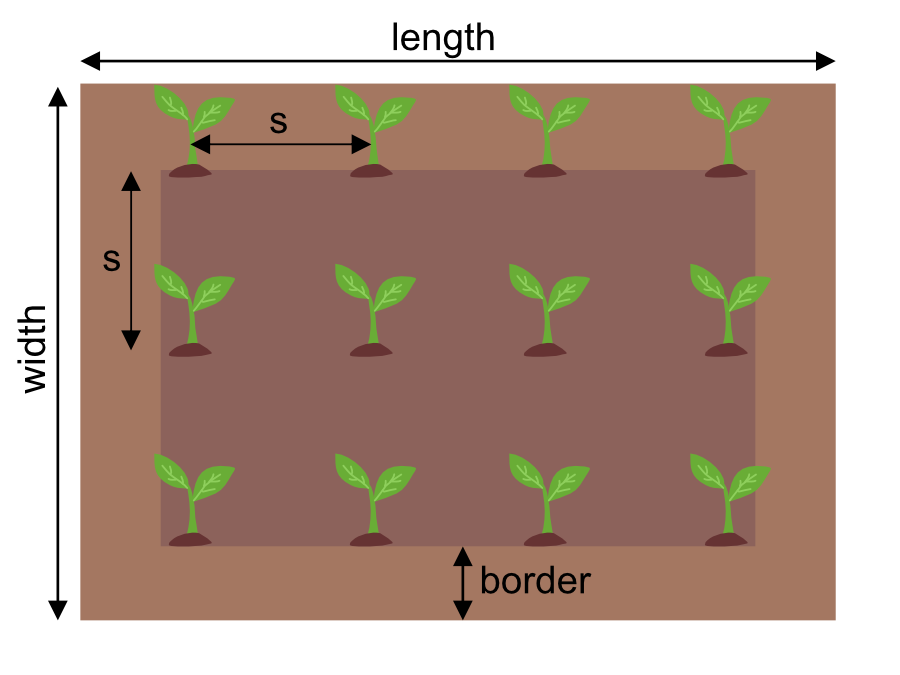Plant grid
Square
Area width
ft
Area length
ft
Area size
ft²
Border
in
Spacing
Plant spacing (s)
in
Density
plants/
ft²
Total plants

# Plant Spacing Calculator

By Bogna Szyk and Jasmine J Mah
Last updated: Mar 16, 2021
Table of contents:

So you want to have an ideal garden, or an ideal orchard, where all of the plants are lined up in perfect rows. The thing is, though... How many plants exactly do you need? With the plant spacing calculator, you will be able to answer this question in a few seconds - regardless of whether you want a square grid, a rectangular grid or triangular spacing, you will be able to calculate the number of plants you can fit in no time at all. Make sure to take a look at the mulch calculator and fertilizer calculator too.

## Square vs. rows vs. triangular spacing

A square grid of plants is fairly simple to understand: you divide the area into squares of equal side length, and put one plant in each corner.

For a rectangular grid (i.e., row planting), you divide the area into rows, and spread plants evenly along each row. Planting in rows means you can have more space between rows and less space between plants within the row. It makes walking between rows easier and has potential benefits for disease management, but isn't as space efficient as triangular spacing.

If you want triangular spacing, you need to create a lattice of equilateral triangles (they all have sides of the same length, and all internal angles are equal to 60 degrees), and place a plant in each corner. This maximizes the use of space, but can have the consequence of diseases spreading more easily in monocrop farming. Triangular spacing is frequently used in landscaping.

## How to use the plant calculator

1. Determine the width and length of the area you want to cover with plants. We will assume an orchard with a width 240 m and a length of 320 m.
2. Determine the width of the border where you won't be putting plants. For our orchard, we will assume the border is 2 m.
3. Decide on the spacing between the plants. Note that this is the spacing between the centers of the plants, so, if you plan for your trees to grow, leave some extra space for it! We will assume spacing of 3.5 m.
4. The plant spacing calculator will tell you how many plants you need in a square or a triangular grid - here, 6,188 for a square grid and 7,059 for triangular spacing.
5. Now simply choose one of the geometry options (square, rectangular, or triangular) and start planting! :)

Notice that you can also use the plant spacing calculator for row spacing so you can have different spacing between rows vs. within a row.

For triangular grids, the calculator will also tell you how far apart to space each row to make the layout easier. For this example, the rows should be spaced 3.03 m apart to result in exactly 3.5 m spacing between each plant in all directions. For triangular rows, sometimes the offset rows (i.e., the "even" rows, if we label the rows 1, 2, 3...) will have one less plant like the example image above the calculator. The plant spacing calculator factors this into the total number of plants.

## What is the formula for plant density?

Sometimes you may be given a target plant density for a particular species (such as 2 plants per m2), and you need to find out the ideal spacing between each plant. The formula to find plant spacing from plant density is different depending on the type of grid.

For a square grid, the formula is:

`plant spacing = √(1 / density)`

and for a triangular grid, the formula is:

`plant spacing = √(2 / (√3 * density))`

where density could be plants/m2 or plants/ft2 and plant spacing could be m or ft, accordingly.

## A fun fact about triangular spacing

Did you know that depending on which way you orient the triangular grid, you might fit a different number of plants in the same area? Sounds bizarre, right?

You can try switching around your numbers for width and length to see how it changes the total number of plants. It makes a lot more sense when you can visualize the triangular grid in the diagram below:

As you can see, turning the triangular grid by 90 degrees actually changes the grid layout, and can therefore result in a different number of plants since a triangular grid is not bisymmetrical. If aesthetics is an important part of your planning, then try playing around with different grid orientations for different effects!

Bogna Szyk and Jasmine J Mah